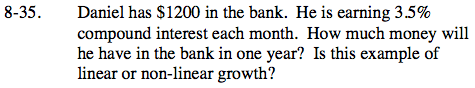Home > ACC7 > Chapter cc38 > Lesson cc38.1.3 > Problem8-35

8-35.

Daniel has \$1200 in the bank. He is earning 3.5% compound interest each month. How much money will he have in the bank in one year? Is this example of linear or non-linear growth? Homework Help ✎${\it A} = {\it P}(1 + {\it r})^{\it n}$

where:
A = total amount including previous interest earned
P = principal
r = interest rate for each compounding period
n = number of time periods

A = 1200(1 + 0.035)12